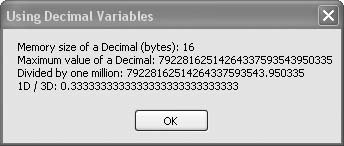# Recipe 6.6. Using Decimal Variables for Maximum Precision

#### Problem

You want to manipulate numbers with many significant digits of accuracy.

#### Solution

Sample code folder: Chapter 06\SingleDouble

The Decimal number type holds numbers with up to 29 digits of accuracy and is well suited to tasks in which rounding errors are to be kept to a minimum, as in financial calculations.

#### Discussion

For really big numbers where you want many digits of accuracy, the Decimal number type is ideal. Numbers of this type are stored in 16 bytes (128 bits) of memory each, with up to 29 significant digits. These numbers can be positive or negative, and a decimal point can be included anywhere within the number. The following code demonstrates Decimal variables in action:

` Dim result As New System.Text.StringBuilder Dim maxDecimal As Decimal = Decimal.MaxValue Dim sizeOfDecimal As Integer = _    Runtime.InteropServices.Marshal.SizeOf(maxDecimal.GetType) result.Append("Memory size of a Decimal (bytes): ") result.AppendLine(sizeOfDecimal) result.Append("Maximum value of a Decimal: ") result.AppendLine(maxDecimal) result.Append("Divided by one million: ") result.AppendLine(maxDecimal / 1000000D) result.Append("1D / 3D: ") result.AppendLine(1D / 3D) MsgBox(result.ToString()) `

Figure 6-6 shows the display created by this code. The Marshal.SizeOf() function determines the number of bytes of memory the Decimal variable uses, and the MaxValue constant gets the largest possible numerical value it can hold. To demonstrate how the decimal point can be anywhere in the number, the maximum value is divided by one million. The decimal point shifts six digits in from the right as a result. To demonstrate that the math operators are overloaded to accurately take advantage of the Decimal's full precision, the quantity 1/3 is calculated and displayed in the last line of the message box. An uppercase "D" is appended to the constants 1 and 3 in the code to tell the compiler that they are Decimal values.

##### Figure 6-6. Using the Decimal number typeVisual Basic 2005 Cookbook: Solutions for VB 2005 Programmers (Cookbooks (OReilly))
ISBN: 0596101775
EAN: 2147483647
Year: 2006
Pages: 400

Similar book on Amazon